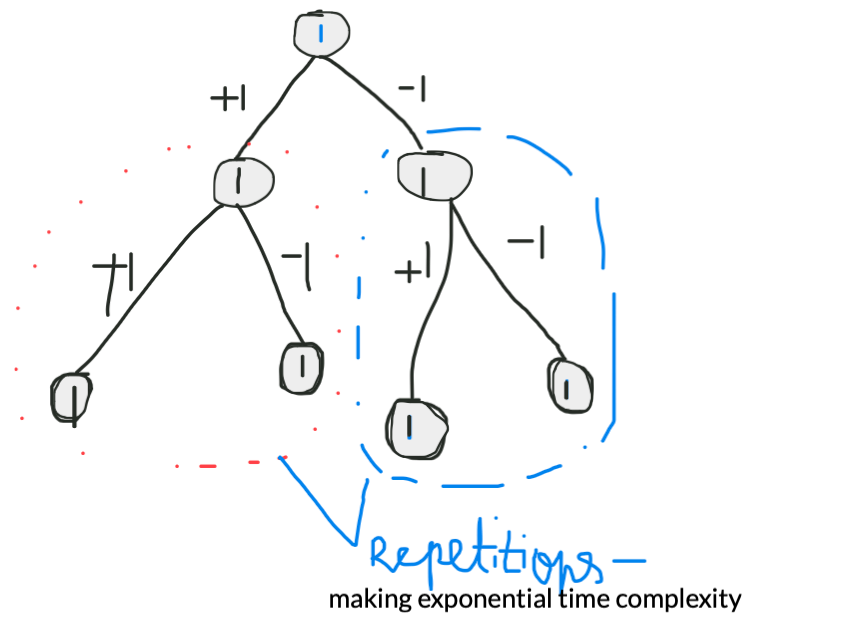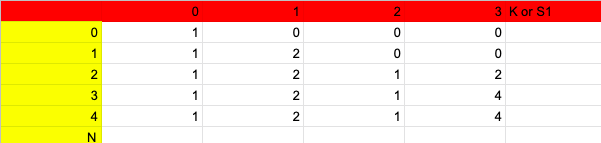×

Search anything:

# Number of expressions that evaluate to value K

#### Algorithms Dynamic Programming (DP)Get this book -> Problems on Array: For Interviews and Competitive Programming

In this problem, we have to form expressions using + and - and find the number of expressions that evaluate to K. This can be solved efficiently using Dynamic Programming and is an extension of Count Subset Problem.

1. Problem Statement
2. Intuition to solve it
3. Steps
4. Brute Force Approach
5. Memorization
6. Using Dynamic Programming
7. Explanation of the logic
8. Furthur optimization?

# Problem Statement

Problem Statement : Given a list of integers and another integer K, place + or - before each integer in the list to form an expression. Find number of expressions that evaluate to value K

Case -1

List =[1,2,3]
K = 6
Output : 1 (+1+2+3=6)
Case -2
List = [1,1,1,1,1]
K=3
Output : +1+1+1+1-1 , +1-1+1+1+1 ... so on

It is observed that we either put + or - before the integer and check if the expression is forming its total value equal to the target . If yes add to the count.

We will see basic approach - recursive then see the memoized version and then DP version

# Intuition to solve it

We perform 2 steps , either subtract the current element or add the current element to K and if K=0 then we increment the answer.

E.g: [1,1,1,1,1] ,K=3

Now there can be various answers:
1+1+1 , 1+1+1+1-1 ,1-1+1+1+1,-1+1+1+1+1 and so on..

We go to each index , experiment with +1 or -1 and check if the rest expression is giving the desired sum i.e K. If yes we add to count.
With firstindex=0
We can choose +1 or -1
With +1 we get +1+1+1 , 1-1+1+1+1 , 1+1-1+1+1,1+1+1-1+1,1+1+1+1-1
With -1 we get -1+1+1+1+1 , this way we keep checking for each index.

# Steps

• Base Case : If the starting index(s) is equal to size of array and k=0 then one expression found. So increment the count.
• Faith - We evaluate for '-' i.e. try subtracting from k and run recursive function.
• Now we evaluate for '+' and try adding nums[s] (current element) to the k and see if any result emanates from this option

# Brute Force Approach

Recursive-

`````` int ans=0;
void find(vector<int>& nums, int k,int s)
{
if(s==nums.size())
{
if(k==0)//if  k-=nums[0..n-1] and k=0 then one expression found!
ans++;

return ;
}
else{
find(nums,k-nums[s],s+1); //Case to put - before the number
find(nums,k+nums[s],s+1); //Case to put + before the number
}

}
int NoOfExpressions(vector<int>& nums, int k) {
int n=nums.size();
find(nums,k,0);
return ans;
}
``````

### Time Complexity

Time complexity for this code is exponential (2^n) due to many unnecessary repetitions happening. Memoization can prevent these repetitions and render polynomial complexity.# Memorization

Since ,the recursive approach renders an exponential time complexity we need to optimize it furthur so that it is optimal. We can do it through memoization. In memoization , we store the computed values for each part. Whenever it is repeated we return the stored ones instead of computing everything again. This saves a lot of time and reduces exponential to polynomial time complexity.

Steps:

• From the recursive function we observe that two parameters change, one is starting index i.e. s and the k(k-nums[s] or k+nums[s])
• We form dp array of array size and k and evaluate and store.
• When same s and k are repeated we return already stored value.
``````vector<unordered_map<int,int>> dp;
int find(vector<int>& nums, int k,int s, vector<unordered_map<int,int>>& dp)
{   int ans=0;
if(s==nums.size())
{
if(k==0)
return 1;

return 0;
}
if(dp[s][k]!=0) return dp[s][k];
//instead of again recursive it returns,thus preventing repetitions

int sub=find(nums,k-nums[s],s+1,dp);

return dp[s][k]=ans;

}
int findTargetSumWays(vector<int>& nums, int k) {
int n=nums.size();
dp=vector<unordered_map<int,int>>(nums.size());
return find(nums,k,0,dp);

}
``````

Time Complexity : O(n * sum)
Space Complexity: O(n * sum)

# Using Dynamic Programming

After certain inferences, we can spot the stark similarity between the "count no of subsets" problem and this.

• Take all the -ve expressioned values to one side and +ve to other.
• It is of the form [+1,+1,+1]+[-1,-1,-1] => [+1,+1,+1] - [1,1,1]
• Let sum of +1+1+1 be S1 and sum of -1-1-1 be S2.
• S1-S2=K --------->(1)
• We know that S1+S2=Total_Sum_Elements(let us denote it as T)---->2
• Therefore 2S1 = T+K----> from adding (1) and (2)
• S1 = (T+K)/2 after furthur simpli
• Now we just need to find the no of subsets with sum = S1.

### Count Subset Problem:

No of Subsets with total {sum = s1}
Recursive Relation
We again have two options , to add current element and to skip it and find number of subsets from both.

``````if(curr==arr.size()) {if(sum==0) count++; return count;}
int including_current_element = rec_func(arr,sum-nums[curr],curr+1)
int excluding_current_element = rec_func(arr,sum,curr+1)
``````

DP StructureTill every element and for respective sum k we calculate number of subsets adding up to k. This is the example of DP table for arr={0,1,2,3,4} and K=3.

We precalculate the 0th row where dp=1 because there is 1 way in without including the element.
for every i from 1 to N:
for every k from 0 to S1:

• We see the previous row if any subset is present of that sum and store it to current computation

• We see if current element at this index which is less or equal to k has any other result?

• If yes we add this to our current computation

Base Case
dp=1 , as to get sum=0 by not adding the current element .

DP[i][j] denotes number of subsets with j sum and till ith element

This is similar to 0/1 knapsack problem where if j>=ARRAY[i-1] then we take the

``````dp[i][j]+=dp[i-1][j]+dp[i-1][j-ARRAY[i-1]]

``````

We either take the previous elements answer (exclude current element) or if the current element subtracted from the current jth sum gives some stored result then we add that too(include the current element).

`````` int findTargetSumWays(vector<int>& nums, int target) {
int sum=0;
for(auto i:nums)
sum+=i; //sum of all elements(T)
if(sum<target or (sum+target)%2!=0) return 0;//not possible if not divisible

int s1=(sum+target)/2;

vector<vector<int>> dp(nums.size()+1,vector<int>(s1+1,0));

dp=1;//no of ways with no elements for s1=0 is 1

for(int i=1;i<=nums.size();i++)
{
for(int j=0;j<=s1;j++)
{
dp[i][j]=dp[i-1][j]; //exclude current element
if(nums[i-1]<=j)
dp[i][j]+=dp[i-1][j-nums[i-1]]; //include current element
}
}
return dp[dp.size()-1][dp.size()-1];//no of subsets with s1 as sum

}
``````

## Explanation of the logic

`````` for(int i=1;i<=nums.size();i++)
{
for(int j=0;j<=s1;j++)
{
dp[i][j]=dp[i-1][j]; //exclude current element
if(nums[i-1]<=j)
dp[i][j]+=dp[i-1][j-nums[i-1]]; //include current element
}
}
``````

In this piece, we either choose to include the current element or exclude it by borrowing the previous result itself.The dp array carries the no of subsets for each target and each element of the input array(list of integers).

e.g. [1,2,3,1,1] and s1=5
possible subsets are: [2,3],[1,2,1,1],[3,1,1],[1,3,1]

lets see for nums i.e. 1.

If we include 1 , then possible subsets would be : [1,2,1,1] and [1,3,1]
If we exclude 1, [2,3],[3,1,1]

• When we exclude the element , we take over the previous value itself to the current result.
• While including 1 (in this case) or any current element , we subtract if from the current s1(j) and we pickup the value of the remainder and add to the current(sub) result.

Time Complexity: O(n * S1) [n=nums.size()]
Space Complexity: O(n * S1) S1: which is the sum we are looking for

# Furthur optimization?

``````if(curr==arr.size()) {if(sum==0) count++; return count;}
int including_current_element = rec_func(arr,sum-nums[curr],curr+1)
int excluding_current_element = rec_func(arr,sum,curr+1)
``````

If we see in recursive relation curr+1 is common to both. So only the sum is changing differently. We may let go of curr+1 dimension and opt for 1-D DP method too.

``````dp = 1
for(int  i : nums)
for(int j=S1;j>=i;j--)
dp[j]+=dp[j-i];

return dp[S1];

``````

Time Complexity O(n* S1) No of elements of array
Space Complexity O(S1) S1: subsets sum :which is the sum we are looking for-----derived from explanation earlier

Now you know how to solve the problem in various ways. Thankyou!
Happy Coding!

#### Rashmitha

Final Year, B.tech (Information Technology ),Indira Gandhi Delhi Technical University For Women

Number of expressions that evaluate to value K